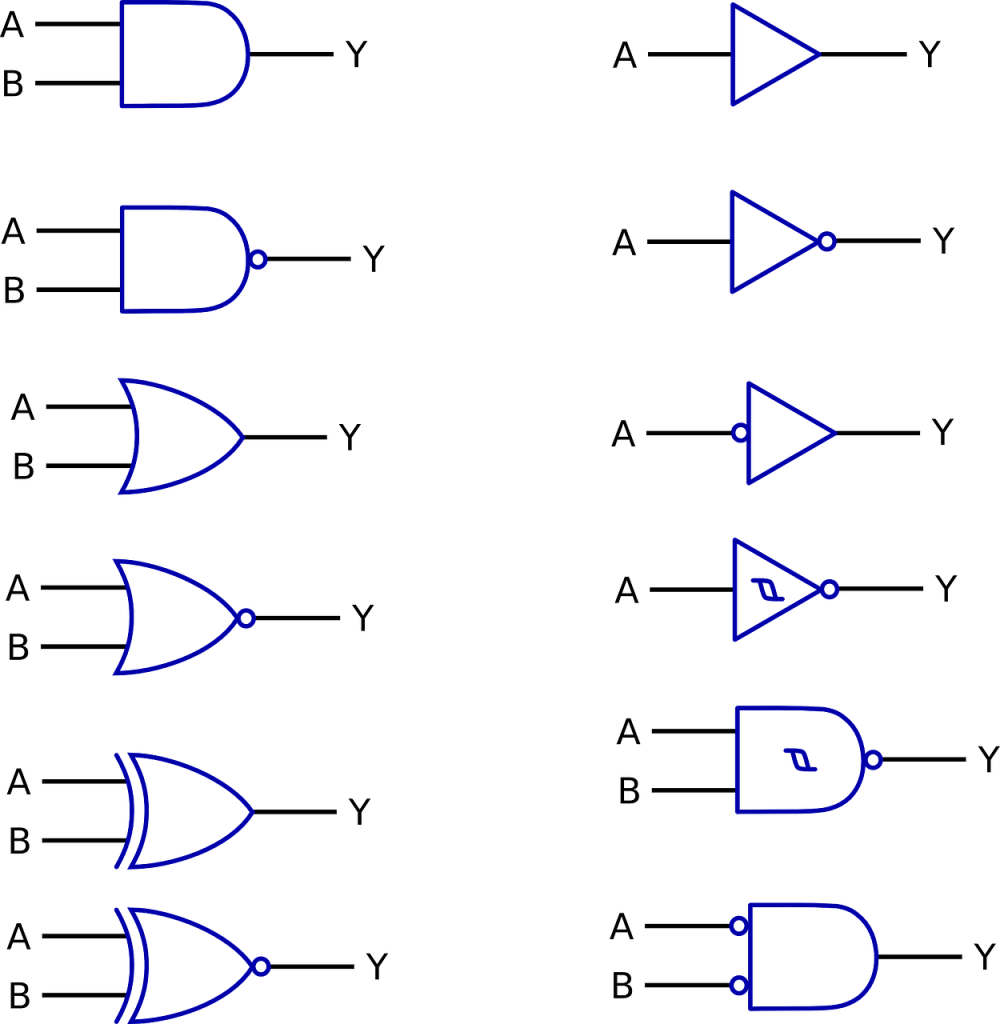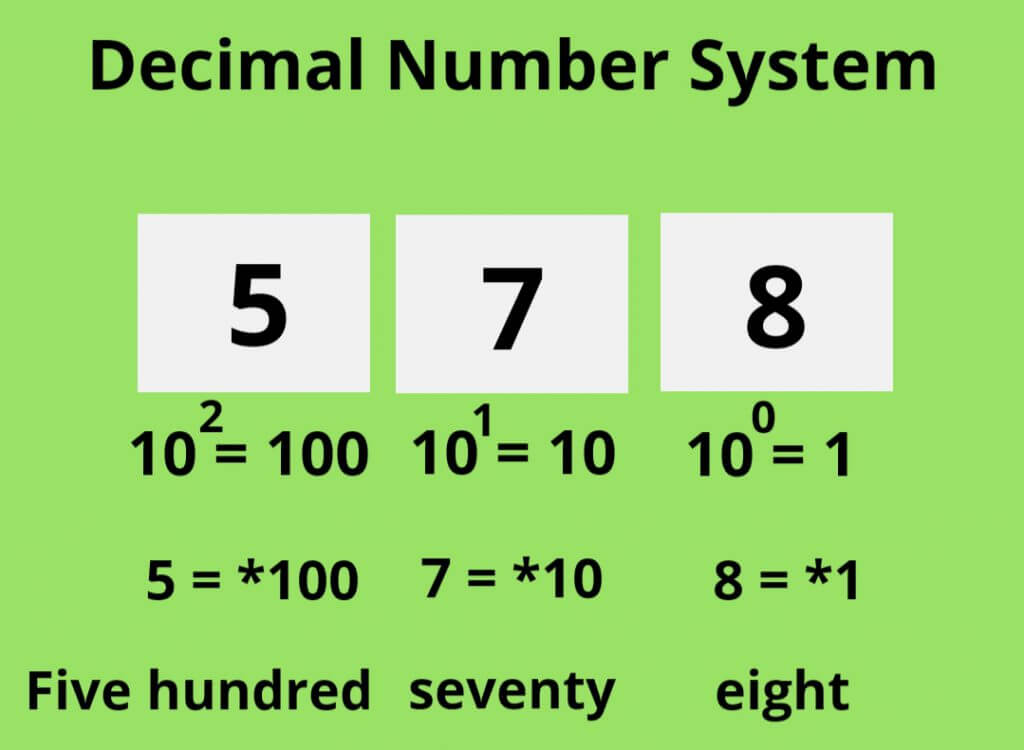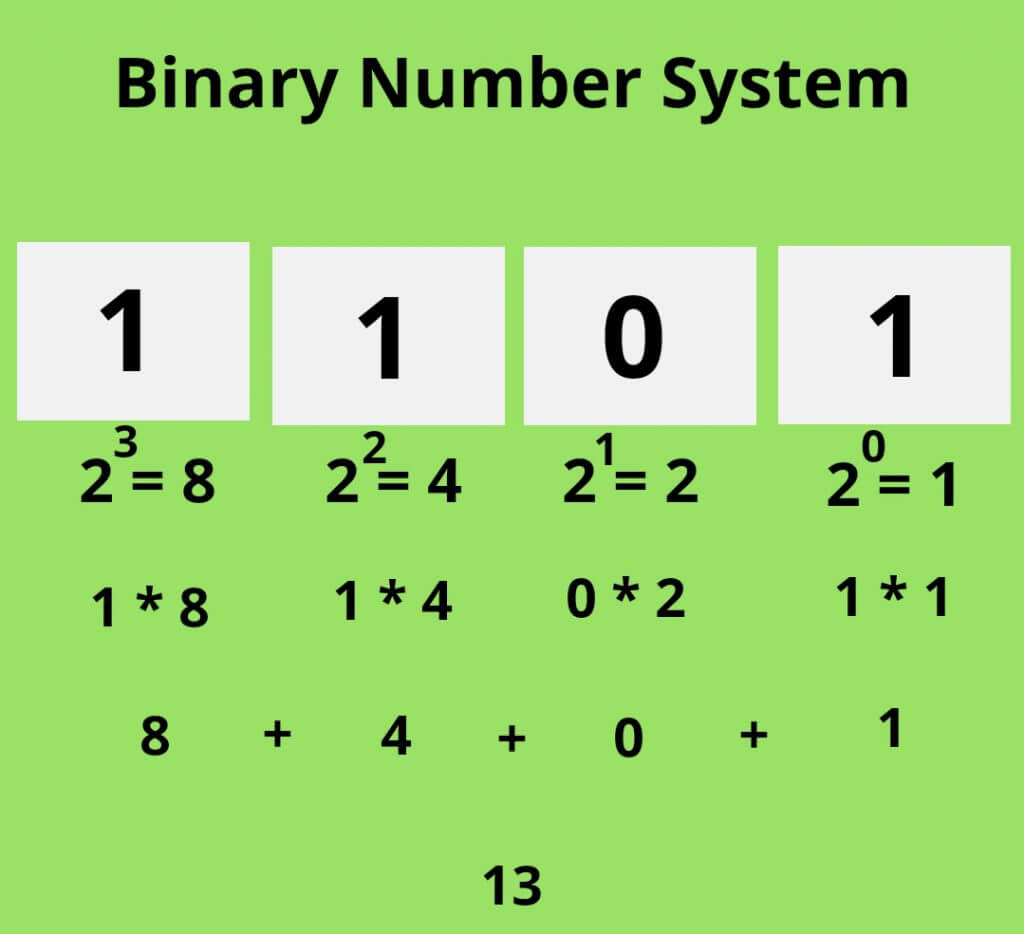How does a Computer Work: Nowadays, we are surrounded by computers which help in performing our day to day work.

Every electronic device you use, for example – Mobile Phone, Microwave, Smartwatch, or even simple Calculation is a Computer.

There are mainly four basic functions of a computer, which is taking Input, Storing it in Memory, Processing the data, and giving the Output.

All these operations are performed with the help of 0s and 1s inside the Computer, which we have discussed below.

We as a human have invented many languages, Like – English, French, Hindi, German, and many more.

These languages help us to communicate and express our thoughts and feelings.

However, do you know what language does computer uses? The language which computer uses is Binary (0 and 1).

Every electronic device, you are using is working on 0 and 1.

You might be wondering that how can a computer get so smart and perform complex tasks and calculations just with the help of 0 and 1.

## Four Basic Functions of a Computer

Every electronic device which can perform these 4 basic functions can be called a Computer.

### 1. Input

A computer consists of Input Devices, like – Keyboard, Mouse, Joystick, and many more, which helps in taking Input from the User.

### 2. Data Storage

Now that Computer has taken Input from the Input Devices. The Data must be stored somewhere (RAM, HDD), so it can be easily retrieved when needed.

### 3. Data Processing

The Data which we have stored in the Storage Unit must be sent to the Processing Unit (CPU) for performing all the Computation on Data to convert it into useful information.

### 4. Output

After the processing of Data, the information gets displayed on Output Devices, like – Monitor, Speaker, Printer, and many more.

## Main Parts of a Computer

There are different parts of a Computer for performing the basic functions. Some of the Main Parts of a Computer are:

### 1. Central Processing Unit (CPU)​

CPU is like the brain of the Computer, which helps in performing Arithmetic, Logical, and Input/Output Operations.

Inside a computer, there is a chip which is called Processor. The processor is an example of a CPU.

### 2. Random Access Memory (RAM)

RAM is the Storage Unit of the Computer, which helps in easy and fast access to the Data.

The data which Computer takes from Input Devices firstly get Stored in RAM. RAM is very fast as compared to other storage devices.

However, the size of RAM is not much and it is used for storing the data temporarily.

### 3. Hard Drive (HDD)

Hard Drive is also the Storage Unit, but it is slow as compared to RAM.

However, the storage capacity of a Hard Drive is very large as compared to RAM. Hard Drive is used for the permanent storage of Data.

### 4. Motherboard

It is the circuit board which provides the platform and connect different components or parts of the computer with each other.

### 5. Video and Audio Card

Video Card is also known as Graphic Card, which helps in handling all the operations related to Graphics.

Without Video Card, you can’t play high-end games on your computer. Similarly, the Audio card is responsible for handling all the operations related to Audio or Sound in a Computer.

Now, You know the basic Functions and Parts of a Computer. So, let’s deep dive into computer architecture to know how does a computer work with 0s and 1s.

## How does a Computer work with 0s and 1s

The computer and all electronic devices, we use works completely based on Binary Code.

When we talk about 1 and 0 in Binary, it refers to ON and OFF state or Yes and No decision, which is taken by the computer.

Everything you see on your computer is done with the help of these two digits(1 or 0) or states(ON or OFF).

Every task you perform on your computer, you get an output in a human-readable form. For example – Text, Image, Video, and many more.

However, inside the hood, every task the computer performs is based on 1 and 0 or Yes and No decision.

If you get deep down on the Computer Architecture, then you will come to know that the computer is just a switch, which can be either turned ON or OFF.

It is just like a bulb that can be either switched ON or OFF.

You might be wondering how the computer can do such incredible tasks with just 1 and 0 or ON and OFF.

The answer is that the computer has over billions of switches inside it, which works simultaneously to perform arithmetic and logical operations.

The brain of the computer which is a Processor have over 15-19 Billion transistors.

The transistors are used to hold and release charges. It can have one of the two states (0 or 1) at a time.

So, if the transistor has a charge then it is said to have a 1 or ON state. And if the transistor has no charge then it is said to have a 0 or OFF state.

With the combination of billions of transistors, the computer can perform complex calculations.

So, you might be thinking that how can computer perform Arithmetic and Logical Operations with just the help of transistors or 0 and 1 state.

The Arithmetic and Logical Operations inside the computer is possible with the help of Logic Gates.These Logic Gates are built with the help of transistors.

Inside the Processor, Transistors are integrated or aligned in such a manner, to form a specific Logic Gate, which ultimately helps in performing calculations.

There are different types of Logic Gates inside the computer, like – OR Gate, AND Gate, NOR Gate, XOR Gate.

With the combination of these Logic Gates, more complex Logic Gates are built which helps in performing Addition, Subtraction, Multiplication, and many more.

These Logic Gates take the input and give the appropriate output.

So, you can also say that Logic Gates is responsible for performing all the operations and calculations on the computer.

## How Computer uses 0s and 1s

We as a human use Decimal Number System which is 0 to 9. Decimal Number System has base 10.

For example, if you take the number 578.Here,
8 have base 10^0 which is 1.
7 have base 10^1 which is 10.
5 have base 10^2 which is 100.

So,
5 = * 100 (Five Hundred)
7 = * 10 (Seventy)
8 = * 1 (Eight)

Therefor, we read it as Five Hundred Seventy Eight.

However, Computer doesn’t understand any other Number System except Binary Number System which is 0 and 1. Binary Number System has base 2.

So, a Binary Number 1101 in the computer is converted in decimal as follows.As the base of the Binary Number System is 2.

So,
1 will have base 2^0 which is 1.
0 will have base 2^1 which is 2.
1 will have base 2^2 which is 4.
1 will have base 2^3 which is 8.

So, the calculation to convert it to decimal will be as follow.

1*8 + 1*4 + 0*2 + 1*0
8 + 4 + 0 + 1
13

So, 1101 in Binary is equal to 13 in Decimal.

Best Course

-> Best Course for learning Python: Python for Everybody Specialization

-> Best Course for learning Java: Java Programming Masterclass covering Java 11 & Java 17

-> Best Course learning JavaScript: The Complete JavaScript Course 2023: From Zero to Expert!

## How does Computer work with Text, Image, and Video

As we know that there is 26 Alphabet in English.

In the computer, each Alphabet or Character is assigned a special ASCII Value.

For example – the ASCII Code of ‘a’ is 097. ASCII Code of ‘A’ is 065. ASCII Code of ‘c’ is 099.

However, these ASCII codes are in Decimal Number System.

As we know the computer doesn’t understand the Decimal Number System, so this ASCII Code is converted into its Binary Equivalent.

So,
a = 097 = 01100001
A = 065 = 01000001
c = 099 = 01100011

So, if you write the text “coderpedia” in your computer, then it will get save in following Binary Code.

01100011 01101111 01100100 01100101 01110010 01110000 01100101 01100100 01101001 01100001

Now let’s talk about how the computer displays the image.

Every photo you see on your monitor is built with the help of several pixels, as the monitor itself is divided into many pixels.

So, a pixel is the smallest unit of an image.

Each pixel has its color. The value of color is in RGB(Red, Green, Blue) format, which is in a human-readable format.

However, the Computer stores these color values in Binary format.

For example – The value of Red Color is (255, 0, 0) and the Binary equivalent of Red color is 111111110000000000000000.

So, each pixel has assigned a specific Binary Code which determines the color of the pixel.

The amount of pixels in the image determines the resolution of your image.

An image is a combination of millions of pixels. So, if the resolution of the image is 2048 * 1536 (weight * height), then the number of pixels in it will be 3,145,728.

The same process is used for video as well.

The only difference is that video is the combination of the number of images in a sequence.

Normally, there are 24 images or frames per second in a video, which is called as frame per second.

So, a sequence of images is displayed to produce a Video.

## High-Level Language to Machine Level Language

If you are a programmer then you might be thinking that if the computer only understands Binary Code, then what is the need for learning High-Level Languages, like – C, C++, Java, or Python.

The answer is that Binary Language is not understandable by humans. So, if someone can’t understand a language, then how can he/she write code or program in it.

Therefore we make use of High-Level Languages (C, C++, Java) for learning programming. However, these languages are not understandable by a computer, as a computer only understands Binary Code.

So, there must be a language that is understandable by humans and close to the computer as well, and therefore, the assembly language is introduced.

Assembly Language is a Low-Level Language that is understandable by humans as well as close to the machine.

Assembly Language is between the High-Level Language (Understandable by humans) and Machine Level Language (Understandable by computers). Therefore, it is called Low-Level Language (Close to humans as well as a computer).

So, when you compile a program which is written in High-Level languages, like – C, C++, Java, then the compiler compiles the program and returns an Assembly Code.

Then that Assembly Code (Low-Level Language) gets converted into the Binary Code (Machine Level Language) with the help of an Assembler.

Finally, the computer gets the Binary Code and it starts performing the execution.

Hope you like the article on How does a Computer work. We also have a article on Linux vs Windows, which you must take a look at.